# Module: measure¶

 Approximate a polygonal chain with the specified tolerance. skimage.measure.block_reduce(image[, …]) Downsample image by applying function func to local blocks. skimage.measure.blur_effect(image[, h_size, …]) Compute a metric that indicates the strength of blur in an image (0 for no blur, 1 for maximal blur). skimage.measure.euler_number(image[, …]) Calculate the Euler characteristic in binary image. skimage.measure.find_contours(image[, …]) Find iso-valued contours in a 2D array for a given level value. skimage.measure.grid_points_in_poly(shape, verts) Test whether points on a specified grid are inside a polygon. skimage.measure.inertia_tensor(image[, mu]) Compute the inertia tensor of the input image. Compute the eigenvalues of the inertia tensor of the image. skimage.measure.label(label_image[, …]) Label connected regions of an integer array. skimage.measure.marching_cubes(volume[, …]) Marching cubes algorithm to find surfaces in 3d volumetric data. skimage.measure.mesh_surface_area(verts, faces) Compute surface area, given vertices & triangular faces skimage.measure.moments(image[, order]) Calculate all raw image moments up to a certain order. skimage.measure.moments_central(image[, …]) Calculate all central image moments up to a certain order. skimage.measure.moments_coords(coords[, order]) Calculate all raw image moments up to a certain order. Calculate all central image moments up to a certain order. Calculate Hu’s set of image moments (2D-only). skimage.measure.moments_normalized(mu[, order]) Calculate all normalized central image moments up to a certain order. skimage.measure.perimeter(image[, neighbourhood]) Calculate total perimeter of all objects in binary image. skimage.measure.perimeter_crofton(image[, …]) Calculate total Crofton perimeter of all objects in binary image. skimage.measure.points_in_poly(points, verts) Test whether points lie inside a polygon. skimage.measure.profile_line(image, src, dst) Return the intensity profile of an image measured along a scan line. skimage.measure.ransac(data, model_class, …) Fit a model to data with the RANSAC (random sample consensus) algorithm. skimage.measure.regionprops(label_image[, …]) Measure properties of labeled image regions. skimage.measure.regionprops_table(label_image) Compute image properties and return them as a pandas-compatible table. skimage.measure.shannon_entropy(image[, base]) Calculate the Shannon entropy of an image. skimage.measure.subdivide_polygon(coords[, …]) Subdivision of polygonal curves using B-Splines. Total least squares estimator for 2D circles. Total least squares estimator for 2D ellipses. Total least squares estimator for N-dimensional lines.

## approximate_polygon¶

skimage.measure.approximate_polygon(coords, tolerance)[source]

Approximate a polygonal chain with the specified tolerance.

It is based on the Douglas-Peucker algorithm.

Note that the approximated polygon is always within the convex hull of the original polygon.

Parameters
coords(N, 2) array

Coordinate array.

tolerancefloat

Maximum distance from original points of polygon to approximated polygonal chain. If tolerance is 0, the original coordinate array is returned.

Returns
coords(M, 2) array

Approximated polygonal chain where M <= N.

References

1

https://en.wikipedia.org/wiki/Ramer-Douglas-Peucker_algorithm

### Examples using skimage.measure.approximate_polygon¶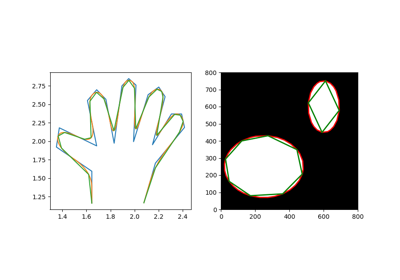## block_reduce¶

skimage.measure.block_reduce(image, block_size=2, func=<function sum>, cval=0, func_kwargs=None)[source]

Downsample image by applying function func to local blocks.

This function is useful for max and mean pooling, for example.

Parameters
imagendarray

N-dimensional input image.

block_sizearray_like or int

Array containing down-sampling integer factor along each axis. Default block_size is 2.

funccallable

Function object which is used to calculate the return value for each local block. This function must implement an axis parameter. Primary functions are numpy.sum, numpy.min, numpy.max, numpy.mean and numpy.median. See also func_kwargs.

cvalfloat

Constant padding value if image is not perfectly divisible by the block size.

func_kwargsdict

Keyword arguments passed to func. Notably useful for passing dtype argument to np.mean. Takes dictionary of inputs, e.g.: func_kwargs={'dtype': np.float16}).

Returns
imagendarray

Down-sampled image with same number of dimensions as input image.

Examples

>>> from skimage.measure import block_reduce
>>> image = np.arange(3*3*4).reshape(3, 3, 4)
>>> image
array([[[ 0,  1,  2,  3],
[ 4,  5,  6,  7],
[ 8,  9, 10, 11]],
[[12, 13, 14, 15],
[16, 17, 18, 19],
[20, 21, 22, 23]],
[[24, 25, 26, 27],
[28, 29, 30, 31],
[32, 33, 34, 35]]])
>>> block_reduce(image, block_size=(3, 3, 1), func=np.mean)
array([[[16., 17., 18., 19.]]])
>>> image_max1 = block_reduce(image, block_size=(1, 3, 4), func=np.max)
>>> image_max1
array([[],
[],
[]])
>>> image_max2 = block_reduce(image, block_size=(3, 1, 4), func=np.max)
>>> image_max2
array([[,
,
]])


## blur_effect¶

skimage.measure.blur_effect(image, h_size=11, channel_axis=None, reduce_func=<function amax>)[source]

Compute a metric that indicates the strength of blur in an image (0 for no blur, 1 for maximal blur).

Parameters
imagendarray

RGB or grayscale nD image. The input image is converted to grayscale before computing the blur metric.

h_sizeint, optional

Size of the re-blurring filter.

channel_axisint or None, optional

If None, the image is assumed to be grayscale (single-channel). Otherwise, this parameter indicates which axis of the array corresponds to color channels.

reduce_funccallable, optional

Function used to calculate the aggregation of blur metrics along all axes. If set to None, the entire list is returned, where the i-th element is the blur metric along the i-th axis.

Returns
blurfloat (0 to 1) or list of floats

Blur metric: by default, the maximum of blur metrics along all axes.

Notes

h_size must keep the same value in order to compare results between images. Most of the time, the default size (11) is enough. This means that the metric can clearly discriminate blur up to an average 11x11 filter; if blur is higher, the metric still gives good results but its values tend towards an asymptote.

References

1

Frederique Crete, Thierry Dolmiere, Patricia Ladret, and Marina Nicolas “The blur effect: perception and estimation with a new no-reference perceptual blur metric” Proc. SPIE 6492, Human Vision and Electronic Imaging XII, 64920I (2007) https://hal.archives-ouvertes.fr/hal-00232709 DOI:10.1117/12.702790

### Examples using skimage.measure.blur_effect¶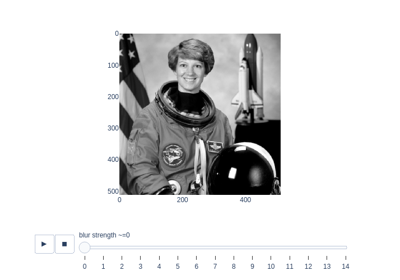## euler_number¶

skimage.measure.euler_number(image, connectivity=None)[source]

Calculate the Euler characteristic in binary image.

For 2D objects, the Euler number is the number of objects minus the number of holes. For 3D objects, the Euler number is obtained as the number of objects plus the number of holes, minus the number of tunnels, or loops.

Parameters
image: (N, M) ndarray or (N, M, D) ndarray.

2D or 3D images. If image is not binary, all values strictly greater than zero are considered as the object.

connectivityint, optional

Maximum number of orthogonal hops to consider a pixel/voxel as a neighbor. Accepted values are ranging from 1 to input.ndim. If None, a full connectivity of input.ndim is used. 4 or 8 neighborhoods are defined for 2D images (connectivity 1 and 2, respectively). 6 or 26 neighborhoods are defined for 3D images, (connectivity 1 and 3, respectively). Connectivity 2 is not defined.

Returns
euler_numberint

Euler characteristic of the set of all objects in the image.

Notes

The Euler characteristic is an integer number that describes the topology of the set of all objects in the input image. If object is 4-connected, then background is 8-connected, and conversely.

The computation of the Euler characteristic is based on an integral geometry formula in discretized space. In practice, a neighbourhood configuration is constructed, and a LUT is applied for each configuration. The coefficients used are the ones of Ohser et al.

It can be useful to compute the Euler characteristic for several connectivities. A large relative difference between results for different connectivities suggests that the image resolution (with respect to the size of objects and holes) is too low.

References

1

S. Rivollier. Analyse d’image geometrique et morphometrique par diagrammes de forme et voisinages adaptatifs generaux. PhD thesis, 2010. Ecole Nationale Superieure des Mines de Saint-Etienne. https://tel.archives-ouvertes.fr/tel-00560838

2

Ohser J., Nagel W., Schladitz K. (2002) The Euler Number of Discretized Sets - On the Choice of Adjacency in Homogeneous Lattices. In: Mecke K., Stoyan D. (eds) Morphology of Condensed Matter. Lecture Notes in Physics, vol 600. Springer, Berlin, Heidelberg.

Examples

>>> import numpy as np
>>> SAMPLE = np.zeros((100,100,100));
>>> SAMPLE[40:60, 40:60, 40:60]=1
>>> euler_number(SAMPLE)
1...
>>> SAMPLE[45:55,45:55,45:55] = 0;
>>> euler_number(SAMPLE)
2...
>>> SAMPLE = np.array([[0, 0, 0, 0, 0, 0, 0, 0, 0, 1, 0, 1, 1, 0, 0, 0, 0, 0],
...                    [0, 0, 0, 0, 0, 0, 0, 0, 1, 1, 1, 1, 0, 0, 0, 0, 0, 0],
...                    [0, 0, 0, 0, 0, 0, 0, 0, 1, 1, 1, 0, 0, 0, 0, 0, 0, 0],
...                    [0, 0, 0, 0, 0, 0, 0, 0, 1, 1, 1, 0, 0, 0, 0, 0, 0, 0],
...                    [0, 0, 0, 0, 0, 0, 1, 1, 1, 1, 1, 1, 0, 0, 0, 0, 0, 0],
...                    [0, 0, 0, 0, 0, 0, 1, 1, 1, 1, 1, 1, 1, 1, 1, 1, 1, 0],
...                    [0, 0, 0, 0, 0, 0, 1, 1, 1, 1, 1, 1, 1, 1, 1, 1, 1, 0],
...                    [1, 0, 1, 0, 0, 1, 1, 0, 1, 1, 0, 0, 1, 1, 1, 1, 1, 0],
...                    [0, 1, 1, 1, 1, 1, 1, 1, 0, 1, 1, 0, 0, 0, 1, 1, 1, 1],
...                    [0, 1, 1, 0, 0, 1, 1, 1, 0, 0, 0, 0, 0, 0, 0, 0, 1, 1]])
>>> euler_number(SAMPLE)  # doctest:
0
>>> euler_number(SAMPLE, connectivity=1)  # doctest:
2


### Examples using skimage.measure.euler_number¶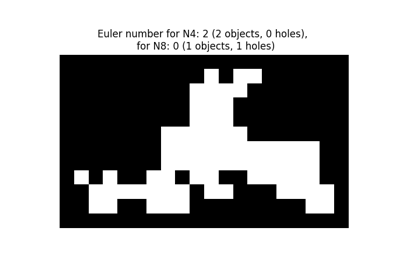## find_contours¶

skimage.measure.find_contours(image, level=None, fully_connected='low', positive_orientation='low', *, mask=None)[source]

Find iso-valued contours in a 2D array for a given level value.

Uses the “marching squares” method to compute a the iso-valued contours of the input 2D array for a particular level value. Array values are linearly interpolated to provide better precision for the output contours.

Parameters
image2D ndarray of double

Input image in which to find contours.

levelfloat, optional

Value along which to find contours in the array. By default, the level is set to (max(image) + min(image)) / 2

Changed in version 0.18: This parameter is now optional.

fully_connectedstr, {‘low’, ‘high’}

Indicates whether array elements below the given level value are to be considered fully-connected (and hence elements above the value will only be face connected), or vice-versa. (See notes below for details.)

positive_orientationstr, {‘low’, ‘high’}

Indicates whether the output contours will produce positively-oriented polygons around islands of low- or high-valued elements. If ‘low’ then contours will wind counter- clockwise around elements below the iso-value. Alternately, this means that low-valued elements are always on the left of the contour. (See below for details.)

mask2D ndarray of bool, or None

A boolean mask, True where we want to draw contours. Note that NaN values are always excluded from the considered region (mask is set to False wherever array is NaN).

Returns
contourslist of (n,2)-ndarrays

Each contour is an ndarray of shape (n, 2), consisting of n (row, column) coordinates along the contour.

Other Parameters
arrayDEPRECATED

Deprecated in favor of image.

Deprecated since version 0.18.

Notes

The marching squares algorithm is a special case of the marching cubes algorithm . A simple explanation is available here:

http://users.polytech.unice.fr/~lingrand/MarchingCubes/algo.html

There is a single ambiguous case in the marching squares algorithm: when a given 2 x 2-element square has two high-valued and two low-valued elements, each pair diagonally adjacent. (Where high- and low-valued is with respect to the contour value sought.) In this case, either the high-valued elements can be ‘connected together’ via a thin isthmus that separates the low-valued elements, or vice-versa. When elements are connected together across a diagonal, they are considered ‘fully connected’ (also known as ‘face+vertex-connected’ or ‘8-connected’). Only high-valued or low-valued elements can be fully-connected, the other set will be considered as ‘face-connected’ or ‘4-connected’. By default, low-valued elements are considered fully-connected; this can be altered with the ‘fully_connected’ parameter.

Output contours are not guaranteed to be closed: contours which intersect the array edge or a masked-off region (either where mask is False or where array is NaN) will be left open. All other contours will be closed. (The closed-ness of a contours can be tested by checking whether the beginning point is the same as the end point.)

Contours are oriented. By default, array values lower than the contour value are to the left of the contour and values greater than the contour value are to the right. This means that contours will wind counter-clockwise (i.e. in ‘positive orientation’) around islands of low-valued pixels. This behavior can be altered with the ‘positive_orientation’ parameter.

The order of the contours in the output list is determined by the position of the smallest x,y (in lexicographical order) coordinate in the contour. This is a side-effect of how the input array is traversed, but can be relied upon.

Warning

Array coordinates/values are assumed to refer to the center of the array element. Take a simple example input: [0, 1]. The interpolated position of 0.5 in this array is midway between the 0-element (at x=0) and the 1-element (at x=1), and thus would fall at x=0.5.

This means that to find reasonable contours, it is best to find contours midway between the expected “light” and “dark” values. In particular, given a binarized array, do not choose to find contours at the low or high value of the array. This will often yield degenerate contours, especially around structures that are a single array element wide. Instead choose a middle value, as above.

References

1

Lorensen, William and Harvey E. Cline. Marching Cubes: A High Resolution 3D Surface Construction Algorithm. Computer Graphics (SIGGRAPH 87 Proceedings) 21(4) July 1987, p. 163-170). DOI:10.1145/37401.37422

Examples

>>> a = np.zeros((3, 3))
>>> a[0, 0] = 1
>>> a
array([[1., 0., 0.],
[0., 0., 0.],
[0., 0., 0.]])
>>> find_contours(a, 0.5)
[array([[0. , 0.5],
[0.5, 0. ]])]


### Examples using skimage.measure.find_contours¶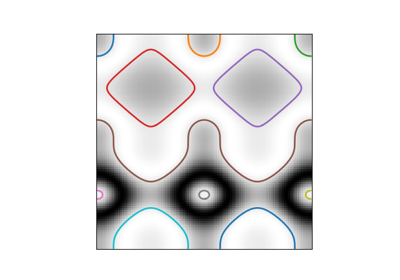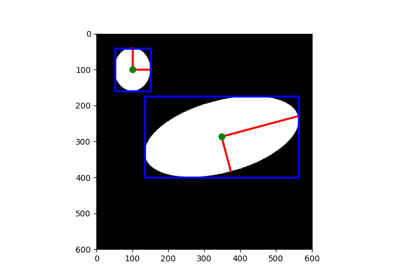## grid_points_in_poly¶

skimage.measure.grid_points_in_poly(shape, verts)[source]

Test whether points on a specified grid are inside a polygon.

For each (r, c) coordinate on a grid, i.e. (0, 0), (0, 1) etc., test whether that point lies inside a polygon.

Parameters
shapetuple (M, N)

Shape of the grid.

verts(V, 2) array

Specify the V vertices of the polygon, sorted either clockwise or anti-clockwise. The first point may (but does not need to be) duplicated.

Returns

True where the grid falls inside the polygon.

## inertia_tensor¶

skimage.measure.inertia_tensor(image, mu=None)[source]

Compute the inertia tensor of the input image.

Parameters
imagearray

The input image.

muarray, optional

The pre-computed central moments of image. The inertia tensor computation requires the central moments of the image. If an application requires both the central moments and the inertia tensor (for example, skimage.measure.regionprops), then it is more efficient to pre-compute them and pass them to the inertia tensor call.

Returns
Tarray, shape (image.ndim, image.ndim)

The inertia tensor of the input image. $$T_{i, j}$$ contains the covariance of image intensity along axes $$i$$ and $$j$$.

References

1

https://en.wikipedia.org/wiki/Moment_of_inertia#Inertia_tensor

2

Bernd Jähne. Spatio-Temporal Image Processing: Theory and Scientific Applications. (Chapter 8: Tensor Methods) Springer, 1993.

## inertia_tensor_eigvals¶

skimage.measure.inertia_tensor_eigvals(image, mu=None, T=None)[source]

Compute the eigenvalues of the inertia tensor of the image.

The inertia tensor measures covariance of the image intensity along the image axes. (See inertia_tensor.) The relative magnitude of the eigenvalues of the tensor is thus a measure of the elongation of a (bright) object in the image.

Parameters
imagearray

The input image.

muarray, optional

The pre-computed central moments of image.

Tarray, shape (image.ndim, image.ndim)

The pre-computed inertia tensor. If T is given, mu and image are ignored.

Returns
eigvalslist of float, length image.ndim

The eigenvalues of the inertia tensor of image, in descending order.

Notes

Computing the eigenvalues requires the inertia tensor of the input image. This is much faster if the central moments (mu) are provided, or, alternatively, one can provide the inertia tensor (T) directly.

## label¶

skimage.measure.label(label_image, background=None, return_num=False, connectivity=None)[source]

Label connected regions of an integer array.

Two pixels are connected when they are neighbors and have the same value. In 2D, they can be neighbors either in a 1- or 2-connected sense. The value refers to the maximum number of orthogonal hops to consider a pixel/voxel a neighbor:

1-connectivity     2-connectivity     diagonal connection close-up

[ ]           [ ]  [ ]  [ ]             [ ]
|               \  |  /                 |  <- hop 2
[ ]--[x]--[ ]      [ ]--[x]--[ ]        [x]--[ ]
|               /  |  \             hop 1
[ ]           [ ]  [ ]  [ ]

Parameters
label_imagendarray of dtype int

Image to label.

backgroundint, optional

Consider all pixels with this value as background pixels, and label them as 0. By default, 0-valued pixels are considered as background pixels.

return_numbool, optional

Whether to return the number of assigned labels.

connectivityint, optional

Maximum number of orthogonal hops to consider a pixel/voxel as a neighbor. Accepted values are ranging from 1 to input.ndim. If None, a full connectivity of input.ndim is used.

Returns
labelsndarray of dtype int

Labeled array, where all connected regions are assigned the same integer value.

numint, optional

Number of labels, which equals the maximum label index and is only returned if return_num is True.

Other Parameters
inputDEPRECATED

Deprecated in favor of label_image.

Deprecated since version 0.19.

References

1

Christophe Fiorio and Jens Gustedt, “Two linear time Union-Find strategies for image processing”, Theoretical Computer Science 154 (1996), pp. 165-181.

2

Kensheng Wu, Ekow Otoo and Arie Shoshani, “Optimizing connected component labeling algorithms”, Paper LBNL-56864, 2005, Lawrence Berkeley National Laboratory (University of California), http://repositories.cdlib.org/lbnl/LBNL-56864

Examples

>>> import numpy as np
>>> x = np.eye(3).astype(int)
>>> print(x)
[[1 0 0]
[0 1 0]
[0 0 1]]
>>> print(label(x, connectivity=1))
[[1 0 0]
[0 2 0]
[0 0 3]]
>>> print(label(x, connectivity=2))
[[1 0 0]
[0 1 0]
[0 0 1]]
>>> print(label(x, background=-1))
[[1 2 2]
[2 1 2]
[2 2 1]]
>>> x = np.array([[1, 0, 0],
...               [1, 1, 5],
...               [0, 0, 0]])
>>> print(label(x))
[[1 0 0]
[1 1 2]
[0 0 0]]


### Examples using skimage.measure.label¶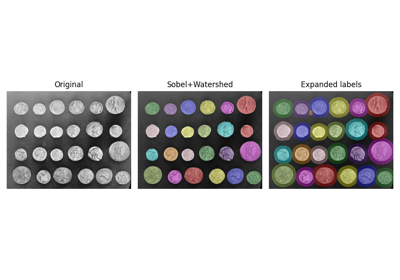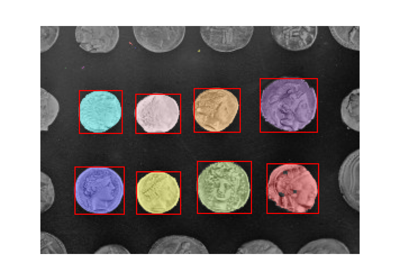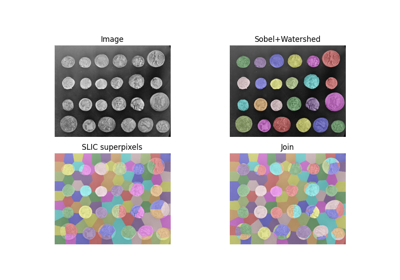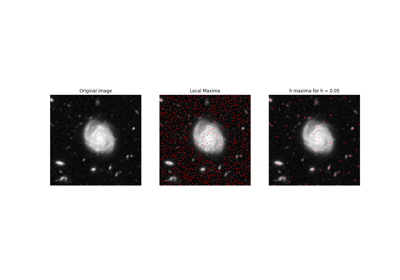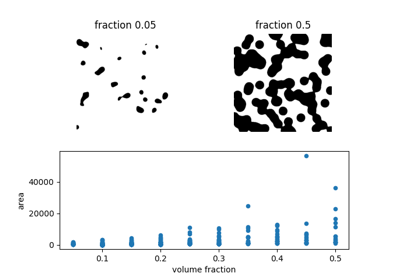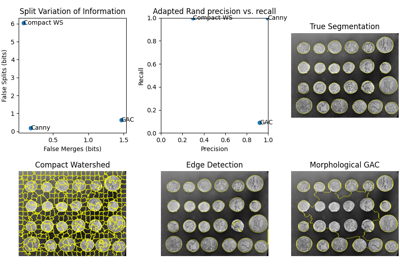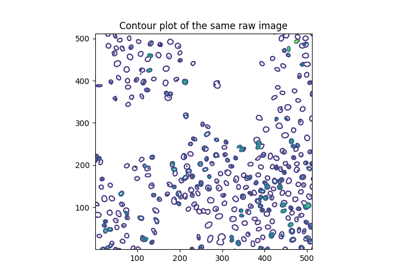## marching_cubes¶

skimage.measure.marching_cubes(volume, level=None, *, spacing=(1.0, 1.0, 1.0), gradient_direction='descent', step_size=1, allow_degenerate=True, method='lewiner', mask=None)[source]

Marching cubes algorithm to find surfaces in 3d volumetric data.

In contrast with Lorensen et al. approach , Lewiner et al. algorithm is faster, resolves ambiguities, and guarantees topologically correct results. Therefore, this algorithm generally a better choice.

Parameters
volume(M, N, P) array

Input data volume to find isosurfaces. Will internally be converted to float32 if necessary.

levelfloat, optional

Contour value to search for isosurfaces in volume. If not given or None, the average of the min and max of vol is used.

spacinglength-3 tuple of floats, optional

Voxel spacing in spatial dimensions corresponding to numpy array indexing dimensions (M, N, P) as in volume.

Controls if the mesh was generated from an isosurface with gradient descent toward objects of interest (the default), or the opposite, considering the left-hand rule. The two options are: * descent : Object was greater than exterior * ascent : Exterior was greater than object

step_sizeint, optional

Step size in voxels. Default 1. Larger steps yield faster but coarser results. The result will always be topologically correct though.

allow_degeneratebool, optional

Whether to allow degenerate (i.e. zero-area) triangles in the end-result. Default True. If False, degenerate triangles are removed, at the cost of making the algorithm slower.

method: str, optional

One of ‘lewiner’, ‘lorensen’ or ‘_lorensen’. Specify which of Lewiner et al. or Lorensen et al. method will be used. The ‘_lorensen’ flag correspond to an old implementation that will be deprecated in version 0.19.

Boolean array. The marching cube algorithm will be computed only on True elements. This will save computational time when interfaces are located within certain region of the volume M, N, P-e.g. the top half of the cube-and also allow to compute finite surfaces-i.e. open surfaces that do not end at the border of the cube.

Returns
verts(V, 3) array

Spatial coordinates for V unique mesh vertices. Coordinate order matches input volume (M, N, P). If allow_degenerate is set to True, then the presence of degenerate triangles in the mesh can make this array have duplicate vertices.

faces(F, 3) array

Define triangular faces via referencing vertex indices from verts. This algorithm specifically outputs triangles, so each face has exactly three indices.

normals(V, 3) array

The normal direction at each vertex, as calculated from the data.

values(V, ) array

Gives a measure for the maximum value of the data in the local region near each vertex. This can be used by visualization tools to apply a colormap to the mesh.

Notes

The algorithm  is an improved version of Chernyaev’s Marching Cubes 33 algorithm. It is an efficient algorithm that relies on heavy use of lookup tables to handle the many different cases, keeping the algorithm relatively easy. This implementation is written in Cython, ported from Lewiner’s C++ implementation.

To quantify the area of an isosurface generated by this algorithm, pass verts and faces to skimage.measure.mesh_surface_area.

Regarding visualization of algorithm output, to contour a volume named myvolume about the level 0.0, using the mayavi package:

>>>
>> from mayavi import mlab
>> verts, faces, _, _ = marching_cubes(myvolume, 0.0)
>> mlab.triangular_mesh([vert for vert in verts],
[vert for vert in verts],
[vert for vert in verts],
faces)
>> mlab.show()


Similarly using the visvis package:

>>>
>> import visvis as vv
>> verts, faces, normals, values = marching_cubes(myvolume, 0.0)
>> vv.mesh(np.fliplr(verts), faces, normals, values)
>> vv.use().Run()


To reduce the number of triangles in the mesh for better performance, see this example using the mayavi package.

References

1

Thomas Lewiner, Helio Lopes, Antonio Wilson Vieira and Geovan Tavares. Efficient implementation of Marching Cubes’ cases with topological guarantees. Journal of Graphics Tools 8(2) pp. 1-15 (december 2003). DOI:10.1080/10867651.2003.10487582

2

Lorensen, William and Harvey E. Cline. Marching Cubes: A High Resolution 3D Surface Construction Algorithm. Computer Graphics (SIGGRAPH 87 Proceedings) 21(4) July 1987, p. 163-170). DOI:10.1145/37401.37422

### Examples using skimage.measure.marching_cubes¶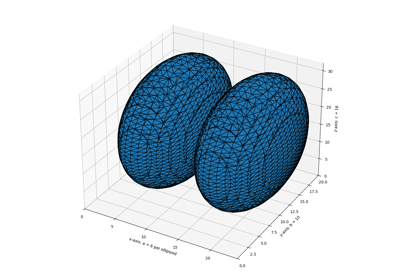## mesh_surface_area¶

skimage.measure.mesh_surface_area(verts, faces)[source]

Compute surface area, given vertices & triangular faces

Parameters
verts(V, 3) array of floats

Array containing (x, y, z) coordinates for V unique mesh vertices.

faces(F, 3) array of ints

List of length-3 lists of integers, referencing vertex coordinates as provided in verts

Returns
areafloat

Surface area of mesh. Units now [coordinate units] ** 2.

skimage.measure.marching_cubes
skimage.measure.marching_cubes_classic

Notes

The arguments expected by this function are the first two outputs from skimage.measure.marching_cubes. For unit correct output, ensure correct spacing was passed to skimage.measure.marching_cubes.

This algorithm works properly only if the faces provided are all triangles.

## moments¶

skimage.measure.moments(image, order=3)[source]

Calculate all raw image moments up to a certain order.

The following properties can be calculated from raw image moments:
• Area as: M[0, 0].

• Centroid as: {M[1, 0] / M[0, 0], M[0, 1] / M[0, 0]}.

Note that raw moments are neither translation, scale nor rotation invariant.

Parameters
imagenD double or uint8 array

Rasterized shape as image.

orderint, optional

Maximum order of moments. Default is 3.

Returns
m(order + 1, order + 1) array

Raw image moments.

References

1

Wilhelm Burger, Mark Burge. Principles of Digital Image Processing: Core Algorithms. Springer-Verlag, London, 2009.

2

B. Jähne. Digital Image Processing. Springer-Verlag, Berlin-Heidelberg, 6. edition, 2005.

3

T. H. Reiss. Recognizing Planar Objects Using Invariant Image Features, from Lecture notes in computer science, p. 676. Springer, Berlin, 1993.

4

https://en.wikipedia.org/wiki/Image_moment

Examples

>>> image = np.zeros((20, 20), dtype=np.double)
>>> image[13:17, 13:17] = 1
>>> M = moments(image)
>>> centroid = (M[1, 0] / M[0, 0], M[0, 1] / M[0, 0])
>>> centroid
(14.5, 14.5)


## moments_central¶

skimage.measure.moments_central(image, center=None, order=3, **kwargs)[source]

Calculate all central image moments up to a certain order.

The center coordinates (cr, cc) can be calculated from the raw moments as: {M[1, 0] / M[0, 0], M[0, 1] / M[0, 0]}.

Note that central moments are translation invariant but not scale and rotation invariant.

Parameters
imagenD double or uint8 array

Rasterized shape as image.

centertuple of float, optional

Coordinates of the image centroid. This will be computed if it is not provided.

orderint, optional

The maximum order of moments computed.

Returns
mu(order + 1, order + 1) array

Central image moments.

References

1

Wilhelm Burger, Mark Burge. Principles of Digital Image Processing: Core Algorithms. Springer-Verlag, London, 2009.

2

B. Jähne. Digital Image Processing. Springer-Verlag, Berlin-Heidelberg, 6. edition, 2005.

3

T. H. Reiss. Recognizing Planar Objects Using Invariant Image Features, from Lecture notes in computer science, p. 676. Springer, Berlin, 1993.

4

https://en.wikipedia.org/wiki/Image_moment

Examples

>>> image = np.zeros((20, 20), dtype=np.double)
>>> image[13:17, 13:17] = 1
>>> M = moments(image)
>>> centroid = (M[1, 0] / M[0, 0], M[0, 1] / M[0, 0])
>>> moments_central(image, centroid)
array([[16.,  0., 20.,  0.],
[ 0.,  0.,  0.,  0.],
[20.,  0., 25.,  0.],
[ 0.,  0.,  0.,  0.]])


## moments_coords¶

skimage.measure.moments_coords(coords, order=3)[source]

Calculate all raw image moments up to a certain order.

The following properties can be calculated from raw image moments:
• Area as: M[0, 0].

• Centroid as: {M[1, 0] / M[0, 0], M[0, 1] / M[0, 0]}.

Note that raw moments are neither translation, scale nor rotation invariant.

Parameters
coords(N, D) double or uint8 array

Array of N points that describe an image of D dimensionality in Cartesian space.

orderint, optional

Maximum order of moments. Default is 3.

Returns
M(order + 1, order + 1, …) array

Raw image moments. (D dimensions)

References

1

Johannes Kilian. Simple Image Analysis By Moments. Durham University, version 0.2, Durham, 2001.

Examples

>>> coords = np.array([[row, col]
...                    for row in range(13, 17)
...                    for col in range(14, 18)], dtype=np.double)
>>> M = moments_coords(coords)
>>> centroid = (M[1, 0] / M[0, 0], M[0, 1] / M[0, 0])
>>> centroid
(14.5, 15.5)


## moments_coords_central¶

skimage.measure.moments_coords_central(coords, center=None, order=3)[source]

Calculate all central image moments up to a certain order.

The following properties can be calculated from raw image moments:
• Area as: M[0, 0].

• Centroid as: {M[1, 0] / M[0, 0], M[0, 1] / M[0, 0]}.

Note that raw moments are neither translation, scale nor rotation invariant.

Parameters
coords(N, D) double or uint8 array

Array of N points that describe an image of D dimensionality in Cartesian space. A tuple of coordinates as returned by np.nonzero is also accepted as input.

centertuple of float, optional

Coordinates of the image centroid. This will be computed if it is not provided.

orderint, optional

Maximum order of moments. Default is 3.

Returns
Mc(order + 1, order + 1, …) array

Central image moments. (D dimensions)

References

1

Johannes Kilian. Simple Image Analysis By Moments. Durham University, version 0.2, Durham, 2001.

Examples

>>> coords = np.array([[row, col]
...                    for row in range(13, 17)
...                    for col in range(14, 18)])
>>> moments_coords_central(coords)
array([[16.,  0., 20.,  0.],
[ 0.,  0.,  0.,  0.],
[20.,  0., 25.,  0.],
[ 0.,  0.,  0.,  0.]])


As seen above, for symmetric objects, odd-order moments (columns 1 and 3, rows 1 and 3) are zero when centered on the centroid, or center of mass, of the object (the default). If we break the symmetry by adding a new point, this no longer holds:

>>> coords2 = np.concatenate((coords, [[17, 17]]), axis=0)
>>> np.round(moments_coords_central(coords2),
...          decimals=2)
array([[17.  ,  0.  , 22.12, -2.49],
[ 0.  ,  3.53,  1.73,  7.4 ],
[25.88,  6.02, 36.63,  8.83],
[ 4.15, 19.17, 14.8 , 39.6 ]])


Image moments and central image moments are equivalent (by definition) when the center is (0, 0):

>>> np.allclose(moments_coords(coords),
...             moments_coords_central(coords, (0, 0)))
True


## moments_hu¶

skimage.measure.moments_hu(nu)[source]

Calculate Hu’s set of image moments (2D-only).

Note that this set of moments is proofed to be translation, scale and rotation invariant.

Parameters
nu(M, M) array

Normalized central image moments, where M must be >= 4.

Returns
nu(7,) array

Hu’s set of image moments.

References

1

M. K. Hu, “Visual Pattern Recognition by Moment Invariants”, IRE Trans. Info. Theory, vol. IT-8, pp. 179-187, 1962

2

Wilhelm Burger, Mark Burge. Principles of Digital Image Processing: Core Algorithms. Springer-Verlag, London, 2009.

3

B. Jähne. Digital Image Processing. Springer-Verlag, Berlin-Heidelberg, 6. edition, 2005.

4

T. H. Reiss. Recognizing Planar Objects Using Invariant Image Features, from Lecture notes in computer science, p. 676. Springer, Berlin, 1993.

5

https://en.wikipedia.org/wiki/Image_moment

Examples

>>> image = np.zeros((20, 20), dtype=np.double)
>>> image[13:17, 13:17] = 0.5
>>> image[10:12, 10:12] = 1
>>> mu = moments_central(image)
>>> nu = moments_normalized(mu)
>>> moments_hu(nu)
array([7.45370370e-01, 3.51165981e-01, 1.04049179e-01, 4.06442107e-02,
2.64312299e-03, 2.40854582e-02, 4.33680869e-19])


## moments_normalized¶

skimage.measure.moments_normalized(mu, order=3)[source]

Calculate all normalized central image moments up to a certain order.

Note that normalized central moments are translation and scale invariant but not rotation invariant.

Parameters
mu(M,[ …,] M) array

Central image moments, where M must be greater than or equal to order.

orderint, optional

Maximum order of moments. Default is 3.

Returns
nu(order + 1,[ …,] order + 1) array

Normalized central image moments.

References

1

Wilhelm Burger, Mark Burge. Principles of Digital Image Processing: Core Algorithms. Springer-Verlag, London, 2009.

2

B. Jähne. Digital Image Processing. Springer-Verlag, Berlin-Heidelberg, 6. edition, 2005.

3

T. H. Reiss. Recognizing Planar Objects Using Invariant Image Features, from Lecture notes in computer science, p. 676. Springer, Berlin, 1993.

4

https://en.wikipedia.org/wiki/Image_moment

Examples

>>> image = np.zeros((20, 20), dtype=np.double)
>>> image[13:17, 13:17] = 1
>>> m = moments(image)
>>> centroid = (m[0, 1] / m[0, 0], m[1, 0] / m[0, 0])
>>> mu = moments_central(image, centroid)
>>> moments_normalized(mu)
array([[       nan,        nan, 0.078125  , 0.        ],
[       nan, 0.        , 0.        , 0.        ],
[0.078125  , 0.        , 0.00610352, 0.        ],
[0.        , 0.        , 0.        , 0.        ]])


## perimeter¶

skimage.measure.perimeter(image, neighbourhood=4)[source]

Calculate total perimeter of all objects in binary image.

Parameters
image(N, M) ndarray

2D binary image.

neighbourhood4 or 8, optional

Neighborhood connectivity for border pixel determination. It is used to compute the contour. A higher neighbourhood widens the border on which the perimeter is computed.

Returns
perimeterfloat

Total perimeter of all objects in binary image.

References

1

K. Benkrid, D. Crookes. Design and FPGA Implementation of a Perimeter Estimator. The Queen’s University of Belfast. http://www.cs.qub.ac.uk/~d.crookes/webpubs/papers/perimeter.doc

Examples

>>> from skimage import data, util
>>> from skimage.measure import label
>>> # coins image (binary)
>>> img_coins = data.coins() > 110
>>> # total perimeter of all objects in the image
>>> perimeter(img_coins, neighbourhood=4)
7796.867...
>>> perimeter(img_coins, neighbourhood=8)
8806.268...


### Examples using skimage.measure.perimeter¶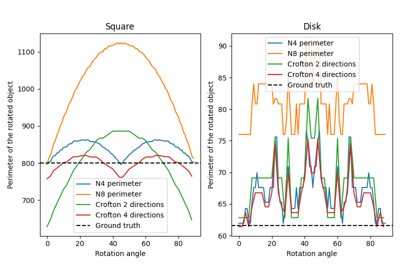## perimeter_crofton¶

skimage.measure.perimeter_crofton(image, directions=4)[source]

Calculate total Crofton perimeter of all objects in binary image.

Parameters
image(N, M) ndarray

2D image. If image is not binary, all values strictly greater than zero are considered as the object.

directions2 or 4, optional

Number of directions used to approximate the Crofton perimeter. By default, 4 is used: it should be more accurate than 2. Computation time is the same in both cases.

Returns
perimeterfloat

Total perimeter of all objects in binary image.

Notes

This measure is based on Crofton formula , which is a measure from integral geometry. It is defined for general curve length evaluation via a double integral along all directions. In a discrete space, 2 or 4 directions give a quite good approximation, 4 being more accurate than 2 for more complex shapes.

Similar to perimeter(), this function returns an approximation of the perimeter in continuous space.

References

1

https://en.wikipedia.org/wiki/Crofton_formula

2

S. Rivollier. Analyse d’image geometrique et morphometrique par diagrammes de forme et voisinages adaptatifs generaux. PhD thesis, 2010. Ecole Nationale Superieure des Mines de Saint-Etienne. https://tel.archives-ouvertes.fr/tel-00560838

Examples

>>> from skimage import data, util
>>> from skimage.measure import label
>>> # coins image (binary)
>>> img_coins = data.coins() > 110
>>> # total perimeter of all objects in the image
>>> perimeter_crofton(img_coins, directions=2)
8144.578...
>>> perimeter_crofton(img_coins, directions=4)
7837.077...


### Examples using skimage.measure.perimeter_crofton¶## points_in_poly¶

skimage.measure.points_in_poly(points, verts)[source]

Test whether points lie inside a polygon.

Parameters
points(N, 2) array

Input points, (x, y).

verts(M, 2) array

Vertices of the polygon, sorted either clockwise or anti-clockwise. The first point may (but does not need to be) duplicated.

Returns

True if corresponding point is inside the polygon.

## profile_line¶

skimage.measure.profile_line(image, src, dst, linewidth=1, order=None, mode='reflect', cval=0.0, *, reduce_func=<function mean>)[source]

Return the intensity profile of an image measured along a scan line.

Parameters
imagendarray, shape (M, N[, C])

The image, either grayscale (2D array) or multichannel (3D array, where the final axis contains the channel information).

srcarray_like, shape (2, )

The coordinates of the start point of the scan line.

dstarray_like, shape (2, )

The coordinates of the end point of the scan line. The destination point is included in the profile, in contrast to standard numpy indexing.

linewidthint, optional

Width of the scan, perpendicular to the line

orderint in {0, 1, 2, 3, 4, 5}, optional

The order of the spline interpolation, default is 0 if image.dtype is bool and 1 otherwise. The order has to be in the range 0-5. See skimage.transform.warp for detail.

mode{‘constant’, ‘nearest’, ‘reflect’, ‘mirror’, ‘wrap’}, optional

How to compute any values falling outside of the image.

cvalfloat, optional

If mode is ‘constant’, what constant value to use outside the image.

reduce_funccallable, optional

Function used to calculate the aggregation of pixel values perpendicular to the profile_line direction when linewidth > 1. If set to None the unreduced array will be returned.

Returns
return_valuearray

The intensity profile along the scan line. The length of the profile is the ceil of the computed length of the scan line.

Examples

>>> x = np.array([[1, 1, 1, 2, 2, 2]])
>>> img = np.vstack([np.zeros_like(x), x, x, x, np.zeros_like(x)])
>>> img
array([[0, 0, 0, 0, 0, 0],
[1, 1, 1, 2, 2, 2],
[1, 1, 1, 2, 2, 2],
[1, 1, 1, 2, 2, 2],
[0, 0, 0, 0, 0, 0]])
>>> profile_line(img, (2, 1), (2, 4))
array([1., 1., 2., 2.])
>>> profile_line(img, (1, 0), (1, 6), cval=4)
array([1., 1., 1., 2., 2., 2., 2.])


The destination point is included in the profile, in contrast to standard numpy indexing. For example:

>>> profile_line(img, (1, 0), (1, 6))  # The final point is out of bounds
array([1., 1., 1., 2., 2., 2., 2.])
>>> profile_line(img, (1, 0), (1, 5))  # This accesses the full first row
array([1., 1., 1., 2., 2., 2.])


For different reduce_func inputs:

>>> profile_line(img, (1, 0), (1, 3), linewidth=3, reduce_func=np.mean)
array([0.66666667, 0.66666667, 0.66666667, 1.33333333])
>>> profile_line(img, (1, 0), (1, 3), linewidth=3, reduce_func=np.max)
array([1, 1, 1, 2])
>>> profile_line(img, (1, 0), (1, 3), linewidth=3, reduce_func=np.sum)
array([2, 2, 2, 4])


The unreduced array will be returned when reduce_func is None or when reduce_func acts on each pixel value individually.

>>> profile_line(img, (1, 2), (4, 2), linewidth=3, order=0,
...     reduce_func=None)
array([[1, 1, 2],
[1, 1, 2],
[1, 1, 2],
[0, 0, 0]])
>>> profile_line(img, (1, 0), (1, 3), linewidth=3, reduce_func=np.sqrt)
array([[1.        , 1.        , 0.        ],
[1.        , 1.        , 0.        ],
[1.        , 1.        , 0.        ],
[1.41421356, 1.41421356, 0.        ]])


## ransac¶

skimage.measure.ransac(data, model_class, min_samples, residual_threshold, is_data_valid=None, is_model_valid=None, max_trials=100, stop_sample_num=inf, stop_residuals_sum=0, stop_probability=1, random_state=None, initial_inliers=None)[source]

Fit a model to data with the RANSAC (random sample consensus) algorithm.

RANSAC is an iterative algorithm for the robust estimation of parameters from a subset of inliers from the complete data set. Each iteration performs the following tasks:

1. Select min_samples random samples from the original data and check whether the set of data is valid (see is_data_valid).

2. Estimate a model to the random subset (model_cls.estimate(*data[random_subset]) and check whether the estimated model is valid (see is_model_valid).

3. Classify all data as inliers or outliers by calculating the residuals to the estimated model (model_cls.residuals(*data)) - all data samples with residuals smaller than the residual_threshold are considered as inliers.

4. Save estimated model as best model if number of inlier samples is maximal. In case the current estimated model has the same number of inliers, it is only considered as the best model if it has less sum of residuals.

These steps are performed either a maximum number of times or until one of the special stop criteria are met. The final model is estimated using all inlier samples of the previously determined best model.

Parameters
data[list, tuple of] (N, …) array

Data set to which the model is fitted, where N is the number of data points and the remaining dimension are depending on model requirements. If the model class requires multiple input data arrays (e.g. source and destination coordinates of skimage.transform.AffineTransform), they can be optionally passed as tuple or list. Note, that in this case the functions estimate(*data), residuals(*data), is_model_valid(model, *random_data) and is_data_valid(*random_data) must all take each data array as separate arguments.

model_classobject

Object with the following object methods:

• success = estimate(*data)

• residuals(*data)

where success indicates whether the model estimation succeeded (True or None for success, False for failure).

min_samplesint in range (0, N)

The minimum number of data points to fit a model to.

residual_thresholdfloat larger than 0

Maximum distance for a data point to be classified as an inlier.

is_data_validfunction, optional

This function is called with the randomly selected data before the model is fitted to it: is_data_valid(*random_data).

is_model_validfunction, optional

This function is called with the estimated model and the randomly selected data: is_model_valid(model, *random_data), .

max_trialsint, optional

Maximum number of iterations for random sample selection.

stop_sample_numint, optional

Stop iteration if at least this number of inliers are found.

stop_residuals_sumfloat, optional

Stop iteration if sum of residuals is less than or equal to this threshold.

stop_probabilityfloat in range [0, 1], optional

RANSAC iteration stops if at least one outlier-free set of the training data is sampled with probability >= stop_probability, depending on the current best model’s inlier ratio and the number of trials. This requires to generate at least N samples (trials):

N >= log(1 - probability) / log(1 - e**m)

where the probability (confidence) is typically set to a high value such as 0.99, e is the current fraction of inliers w.r.t. the total number of samples, and m is the min_samples value.

random_state{None, int, numpy.random.Generator}, optional

If random_state is None the numpy.random.Generator singleton is used. If random_state is an int, a new Generator instance is used, seeded with random_state. If random_state is already a Generator instance then that instance is used.

initial_inliersarray-like of bool, shape (N,), optional

Initial samples selection for model estimation

Returns
modelobject

Best model with largest consensus set.

inliers(N, ) array

Boolean mask of inliers classified as True.

References

1

“RANSAC”, Wikipedia, https://en.wikipedia.org/wiki/RANSAC

Examples

Generate ellipse data without tilt and add noise:

>>> t = np.linspace(0, 2 * np.pi, 50)
>>> xc, yc = 20, 30
>>> a, b = 5, 10
>>> x = xc + a * np.cos(t)
>>> y = yc + b * np.sin(t)
>>> data = np.column_stack([x, y])
>>> rng = np.random.default_rng(203560)  # do not copy this value
>>> data += rng.normal(size=data.shape)


>>> data = (100, 100)
>>> data = (110, 120)
>>> data = (120, 130)
>>> data = (140, 130)


Estimate ellipse model using all available data:

>>> model = EllipseModel()
>>> model.estimate(data)
True
>>> np.round(model.params)
array([ 72.,  75.,  77.,  14.,   1.])


Estimate ellipse model using RANSAC:

>>> ransac_model, inliers = ransac(data, EllipseModel, 20, 3, max_trials=50)
>>> abs(np.round(ransac_model.params))
array([20., 30., 10.,  6.,  2.])
>>> inliers
array([False, False, False, False,  True,  True,  True,  True,  True,
True,  True,  True,  True,  True,  True,  True,  True,  True,
True,  True,  True,  True,  True,  True,  True,  True,  True,
True,  True,  True,  True,  True,  True,  True,  True,  True,
True,  True,  True,  True,  True,  True,  True,  True,  True,
True,  True,  True,  True,  True], dtype=bool)
>>> sum(inliers) > 40
True


RANSAC can be used to robustly estimate a geometric transformation. In this section, we also show how to use a proportion of the total samples, rather than an absolute number.

>>> from skimage.transform import SimilarityTransform
>>> rng = np.random.default_rng()
>>> src = 100 * rng.random((50, 2))
>>> model0 = SimilarityTransform(scale=0.5, rotation=1,
...                              translation=(10, 20))
>>> dst = model0(src)
>>> dst = (10000, 10000)
>>> dst = (-100, 100)
>>> dst = (50, 50)
>>> ratio = 0.5  # use half of the samples
>>> min_samples = int(ratio * len(src))
>>> model, inliers = ransac((src, dst), SimilarityTransform, min_samples,
...                         10,
...                         initial_inliers=np.ones(len(src), dtype=bool))
>>> inliers
array([False, False, False,  True,  True,  True,  True,  True,  True,
True,  True,  True,  True,  True,  True,  True,  True,  True,
True,  True,  True,  True,  True,  True,  True,  True,  True,
True,  True,  True,  True,  True,  True,  True,  True,  True,
True,  True,  True,  True,  True,  True,  True,  True,  True,
True,  True,  True,  True,  True])


### Examples using skimage.measure.ransac¶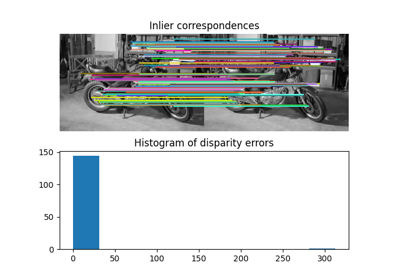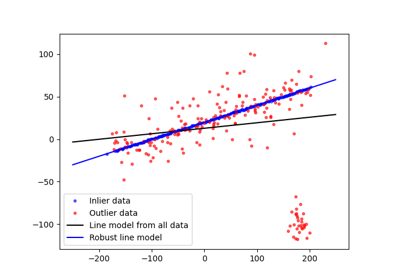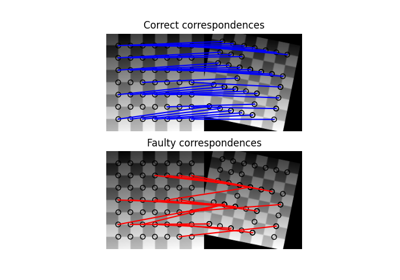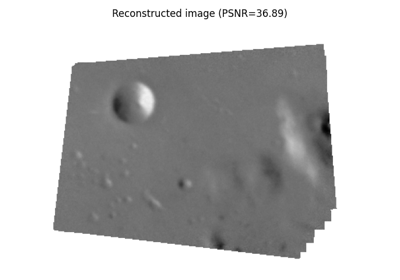## regionprops¶

skimage.measure.regionprops(label_image, intensity_image=None, cache=True, coordinates=None, *, extra_properties=None)[source]

Measure properties of labeled image regions.

Parameters
label_image(M, N[, P]) ndarray

Labeled input image. Labels with value 0 are ignored.

Changed in version 0.14.1: Previously, label_image was processed by numpy.squeeze and so any number of singleton dimensions was allowed. This resulted in inconsistent handling of images with singleton dimensions. To recover the old behaviour, use regionprops(np.squeeze(label_image), ...).

intensity_image(M, N[, P][, C]) ndarray, optional

Intensity (i.e., input) image with same size as labeled image, plus optionally an extra dimension for multichannel data. Currently, this extra channel dimension, if present, must be the last axis. Default is None.

Changed in version 0.18.0: The ability to provide an extra dimension for channels was added.

cachebool, optional

Determine whether to cache calculated properties. The computation is much faster for cached properties, whereas the memory consumption increases.

coordinatesDEPRECATED

This argument is deprecated and will be removed in a future version of scikit-image.

See Coordinate conventions for more details.

Deprecated since version 0.16.0: Use “rc” coordinates everywhere. It may be sufficient to call numpy.transpose on your label image to get the same values as 0.15 and earlier. However, for some properties, the transformation will be less trivial. For example, the new orientation is $$\frac{\pi}{2}$$ plus the old orientation.

extra_propertiesIterable of callables

Add extra property computation functions that are not included with skimage. The name of the property is derived from the function name, the dtype is inferred by calling the function on a small sample. If the name of an extra property clashes with the name of an existing property the extra property wil not be visible and a UserWarning is issued. A property computation function must take a region mask as its first argument. If the property requires an intensity image, it must accept the intensity image as the second argument.

Returns
propertieslist of RegionProperties

Each item describes one labeled region, and can be accessed using the attributes listed below.

Notes

The following properties can be accessed as attributes or keys:

areaint

Number of pixels of the region.

area_bboxint

Number of pixels of bounding box.

area_convexint

Number of pixels of convex hull image, which is the smallest convex polygon that encloses the region.

area_filledint

Number of pixels of the region will all the holes filled in. Describes the area of the image_filled.

axis_major_lengthfloat

The length of the major axis of the ellipse that has the same normalized second central moments as the region.

axis_minor_lengthfloat

The length of the minor axis of the ellipse that has the same normalized second central moments as the region.

bboxtuple

Bounding box (min_row, min_col, max_row, max_col). Pixels belonging to the bounding box are in the half-open interval [min_row; max_row) and [min_col; max_col).

centroidarray

Centroid coordinate tuple (row, col).

centroid_localarray

Centroid coordinate tuple (row, col), relative to region bounding box.

centroid_weightedarray

Centroid coordinate tuple (row, col) weighted with intensity image.

centroid_weighted_localarray

Centroid coordinate tuple (row, col), relative to region bounding box, weighted with intensity image.

coords(N, 2) ndarray

Coordinate list (row, col) of the region.

eccentricityfloat

Eccentricity of the ellipse that has the same second-moments as the region. The eccentricity is the ratio of the focal distance (distance between focal points) over the major axis length. The value is in the interval [0, 1). When it is 0, the ellipse becomes a circle.

equivalent_diameter_areafloat

The diameter of a circle with the same area as the region.

euler_numberint

Euler characteristic of the set of non-zero pixels. Computed as number of connected components subtracted by number of holes (input.ndim connectivity). In 3D, number of connected components plus number of holes subtracted by number of tunnels.

extentfloat

Ratio of pixels in the region to pixels in the total bounding box. Computed as area / (rows * cols)

feret_diameter_maxfloat

Maximum Feret’s diameter computed as the longest distance between points around a region’s convex hull contour as determined by find_contours. 

image(H, J) ndarray

Sliced binary region image which has the same size as bounding box.

image_convex(H, J) ndarray

Binary convex hull image which has the same size as bounding box.

image_filled(H, J) ndarray

Binary region image with filled holes which has the same size as bounding box.

image_intensityndarray

Image inside region bounding box.

inertia_tensorndarray

Inertia tensor of the region for the rotation around its mass.

inertia_tensor_eigvalstuple

The eigenvalues of the inertia tensor in decreasing order.

intensity_maxfloat

Value with the greatest intensity in the region.

intensity_meanfloat

Value with the mean intensity in the region.

intensity_minfloat

Value with the least intensity in the region.

labelint

The label in the labeled input image.

moments(3, 3) ndarray

Spatial moments up to 3rd order:

m_ij = sum{ array(row, col) * row^i * col^j }


where the sum is over the row, col coordinates of the region.

moments_central(3, 3) ndarray

Central moments (translation invariant) up to 3rd order:

mu_ij = sum{ array(row, col) * (row - row_c)^i * (col - col_c)^j }


where the sum is over the row, col coordinates of the region, and row_c and col_c are the coordinates of the region’s centroid.

moments_hutuple

Hu moments (translation, scale and rotation invariant).

moments_normalized(3, 3) ndarray

Normalized moments (translation and scale invariant) up to 3rd order:

nu_ij = mu_ij / m_00^[(i+j)/2 + 1]


where m_00 is the zeroth spatial moment.

moments_weighted(3, 3) ndarray

Spatial moments of intensity image up to 3rd order:

wm_ij = sum{ array(row, col) * row^i * col^j }


where the sum is over the row, col coordinates of the region.

moments_weighted_central(3, 3) ndarray

Central moments (translation invariant) of intensity image up to 3rd order:

wmu_ij = sum{ array(row, col) * (row - row_c)^i * (col - col_c)^j }


where the sum is over the row, col coordinates of the region, and row_c and col_c are the coordinates of the region’s weighted centroid.

moments_weighted_hutuple

Hu moments (translation, scale and rotation invariant) of intensity image.

moments_weighted_normalized(3, 3) ndarray

Normalized moments (translation and scale invariant) of intensity image up to 3rd order:

wnu_ij = wmu_ij / wm_00^[(i+j)/2 + 1]


where wm_00 is the zeroth spatial moment (intensity-weighted area).

orientationfloat

Angle between the 0th axis (rows) and the major axis of the ellipse that has the same second moments as the region, ranging from -pi/2 to pi/2 counter-clockwise.

perimeterfloat

Perimeter of object which approximates the contour as a line through the centers of border pixels using a 4-connectivity.

perimeter_croftonfloat

Perimeter of object approximated by the Crofton formula in 4 directions.

slicetuple of slices

A slice to extract the object from the source image.

solidityfloat

Ratio of pixels in the region to pixels of the convex hull image.

Each region also supports iteration, so that you can do:

for prop in region:
print(prop, region[prop])


References

1

Wilhelm Burger, Mark Burge. Principles of Digital Image Processing: Core Algorithms. Springer-Verlag, London, 2009.

2

B. Jähne. Digital Image Processing. Springer-Verlag, Berlin-Heidelberg, 6. edition, 2005.

3

T. H. Reiss. Recognizing Planar Objects Using Invariant Image Features, from Lecture notes in computer science, p. 676. Springer, Berlin, 1993.

4

https://en.wikipedia.org/wiki/Image_moment

5

W. Pabst, E. Gregorová. Characterization of particles and particle systems, pp. 27-28. ICT Prague, 2007. https://old.vscht.cz/sil/keramika/Characterization_of_particles/CPPS%20_English%20version_.pdf

Examples

>>> from skimage import data, util
>>> from skimage.measure import label, regionprops
>>> img = util.img_as_ubyte(data.coins()) > 110
>>> label_img = label(img, connectivity=img.ndim)
>>> props = regionprops(label_img)
>>> # centroid of first labeled object
>>> props.centroid
(22.72987986048314, 81.91228523446583)
>>> # centroid of first labeled object
>>> props['centroid']
(22.72987986048314, 81.91228523446583)


Add custom measurements by passing functions as extra_properties

>>> from skimage import data, util
>>> from skimage.measure import label, regionprops
>>> import numpy as np
>>> img = util.img_as_ubyte(data.coins()) > 110
>>> label_img = label(img, connectivity=img.ndim)
>>> props = regionprops(label_img, extra_properties=(pixelcount,))
>>> props.pixelcount
7741
>>> props['pixelcount']
42


### Examples using skimage.measure.regionprops¶## regionprops_table¶

skimage.measure.regionprops_table(label_image, intensity_image=None, properties=('label', 'bbox'), *, cache=True, separator='-', extra_properties=None)[source]

Compute image properties and return them as a pandas-compatible table.

The table is a dictionary mapping column names to value arrays. See Notes section below for details.

New in version 0.16.

Parameters
label_image(N, M[, P]) ndarray

Labeled input image. Labels with value 0 are ignored.

intensity_image(M, N[, P][, C]) ndarray, optional

Intensity (i.e., input) image with same size as labeled image, plus optionally an extra dimension for multichannel data. Currently, this extra channel dimension, if present, must be the last axis. Default is None.

Changed in version 0.18.0: The ability to provide an extra dimension for channels was added.

propertiestuple or list of str, optional

Properties that will be included in the resulting dictionary For a list of available properties, please see regionprops(). Users should remember to add “label” to keep track of region identities.

cachebool, optional

Determine whether to cache calculated properties. The computation is much faster for cached properties, whereas the memory consumption increases.

separatorstr, optional

For non-scalar properties not listed in OBJECT_COLUMNS, each element will appear in its own column, with the index of that element separated from the property name by this separator. For example, the inertia tensor of a 2D region will appear in four columns: inertia_tensor-0-0, inertia_tensor-0-1, inertia_tensor-1-0, and inertia_tensor-1-1 (where the separator is -).

Object columns are those that cannot be split in this way because the number of columns would change depending on the object. For example, image and coords.

extra_propertiesIterable of callables

Add extra property computation functions that are not included with skimage. The name of the property is derived from the function name, the dtype is inferred by calling the function on a small sample. If the name of an extra property clashes with the name of an existing property the extra property wil not be visible and a UserWarning is issued. A property computation function must take a region mask as its first argument. If the property requires an intensity image, it must accept the intensity image as the second argument.

Returns
out_dictdict

Dictionary mapping property names to an array of values of that property, one value per region. This dictionary can be used as input to pandas DataFrame to map property names to columns in the frame and regions to rows. If the image has no regions, the arrays will have length 0, but the correct type.

Notes

Each column contains either a scalar property, an object property, or an element in a multidimensional array.

Properties with scalar values for each region, such as “eccentricity”, will appear as a float or int array with that property name as key.

Multidimensional properties of fixed size for a given image dimension, such as “centroid” (every centroid will have three elements in a 3D image, no matter the region size), will be split into that many columns, with the name {property_name}{separator}{element_num} (for 1D properties), {property_name}{separator}{elem_num0}{separator}{elem_num1} (for 2D properties), and so on.

For multidimensional properties that don’t have a fixed size, such as “image” (the image of a region varies in size depending on the region size), an object array will be used, with the corresponding property name as the key.

Examples

>>> from skimage import data, util, measure
>>> image = data.coins()
>>> label_image = measure.label(image > 110, connectivity=image.ndim)
>>> props = measure.regionprops_table(label_image, image,
...                           properties=['label', 'inertia_tensor',
...                                       'inertia_tensor_eigvals'])
>>> props
{'label': array([ 1,  2, ...]), ...
'inertia_tensor-0-0': array([  4.012...e+03,   8.51..., ...]), ...
...,
'inertia_tensor_eigvals-1': array([  2.67...e+02,   2.83..., ...])}


The resulting dictionary can be directly passed to pandas, if installed, to obtain a clean DataFrame:

>>> import pandas as pd
>>> data = pd.DataFrame(props)
label  inertia_tensor-0-0  ...  inertia_tensor_eigvals-1
0      1         4012.909888  ...                267.065503
1      2            8.514739  ...                  2.834806
2      3            0.666667  ...                  0.000000
3      4            0.000000  ...                  0.000000
4      5            0.222222  ...                  0.111111


[5 rows x 7 columns]

If we want to measure a feature that does not come as a built-in property, we can define custom functions and pass them as extra_properties. For example, we can create a custom function that measures the intensity quartiles in a region:

>>> from skimage import data, util, measure
>>> import numpy as np
...     return np.percentile(intensity[regionmask], q=(25, 50, 75))
>>>
>>> image = data.coins()
>>> label_image = measure.label(image > 110, connectivity=image.ndim)
>>> props = measure.regionprops_table(label_image, intensity_image=image,
...                                   properties=('label',),
...                                   extra_properties=(quartiles,))
>>> import pandas as pd
label  quartiles-0  quartiles-1  quartiles-2
0      1       117.00        123.0        130.0
1      2       111.25        112.0        114.0
2      3       111.00        111.0        111.0
3      4       111.00        111.5        112.5
4      5       112.50        113.0        114.0


### Examples using skimage.measure.regionprops_table¶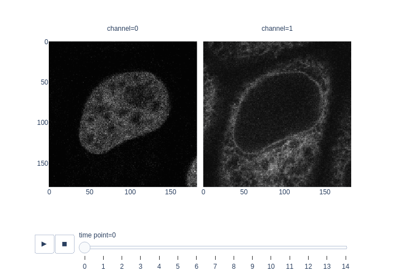## shannon_entropy¶

skimage.measure.shannon_entropy(image, base=2)[source]

Calculate the Shannon entropy of an image.

The Shannon entropy is defined as S = -sum(pk * log(pk)), where pk are frequency/probability of pixels of value k.

Parameters
image(N, M) ndarray

Grayscale input image.

basefloat, optional

The logarithmic base to use.

Returns
entropyfloat

Notes

The returned value is measured in bits or shannon (Sh) for base=2, natural unit (nat) for base=np.e and hartley (Hart) for base=10.

References

1

https://en.wikipedia.org/wiki/Entropy_(information_theory)

2

https://en.wiktionary.org/wiki/Shannon_entropy

Examples

>>> from skimage import data
>>> from skimage.measure import shannon_entropy
>>> shannon_entropy(data.camera())
7.231695011055706


## subdivide_polygon¶

skimage.measure.subdivide_polygon(coords, degree=2, preserve_ends=False)[source]

Subdivision of polygonal curves using B-Splines.

Note that the resulting curve is always within the convex hull of the original polygon. Circular polygons stay closed after subdivision.

Parameters
coords(N, 2) array

Coordinate array.

degree{1, 2, 3, 4, 5, 6, 7}, optional

Degree of B-Spline. Default is 2.

preserve_endsbool, optional

Preserve first and last coordinate of non-circular polygon. Default is False.

Returns
coords(M, 2) array

Subdivided coordinate array.

References

1

http://mrl.nyu.edu/publications/subdiv-course2000/coursenotes00.pdf

### Examples using skimage.measure.subdivide_polygon¶## CircleModel¶

class skimage.measure.CircleModel[source]

Bases: skimage.measure.fit.BaseModel

Total least squares estimator for 2D circles.

The functional model of the circle is:

r**2 = (x - xc)**2 + (y - yc)**2


This estimator minimizes the squared distances from all points to the circle:

min{ sum((r - sqrt((x_i - xc)**2 + (y_i - yc)**2))**2) }


A minimum number of 3 points is required to solve for the parameters.

Notes

The estimation is carried out using a 2D version of the spherical estimation given in .

References

1

Jekel, Charles F. Obtaining non-linear orthotropic material models for pvc-coated polyester via inverse bubble inflation. Thesis (MEng), Stellenbosch University, 2016. Appendix A, pp. 83-87. https://hdl.handle.net/10019.1/98627

Examples

>>> t = np.linspace(0, 2 * np.pi, 25)
>>> xy = CircleModel().predict_xy(t, params=(2, 3, 4))
>>> model = CircleModel()
>>> model.estimate(xy)
True
>>> tuple(np.round(model.params, 5))
(2.0, 3.0, 4.0)
>>> res = model.residuals(xy)
>>> np.abs(np.round(res, 9))
array([0., 0., 0., 0., 0., 0., 0., 0., 0., 0., 0., 0., 0., 0., 0., 0., 0.,
0., 0., 0., 0., 0., 0., 0., 0.])

Attributes
paramstuple

Circle model parameters in the following order xc, yc, r.

__init__()[source]

Initialize self. See help(type(self)) for accurate signature.

estimate(data)[source]

Estimate circle model from data using total least squares.

Parameters
data(N, 2) array

N points with (x, y) coordinates, respectively.

Returns
successbool

True, if model estimation succeeds.

predict_xy(t, params=None)[source]

Predict x- and y-coordinates using the estimated model.

Parameters
tarray

Angles in circle in radians. Angles start to count from positive x-axis to positive y-axis in a right-handed system.

params(3, ) array, optional

Optional custom parameter set.

Returns
xy(…, 2) array

Predicted x- and y-coordinates.

residuals(data)[source]

Determine residuals of data to model.

For each point the shortest distance to the circle is returned.

Parameters
data(N, 2) array

N points with (x, y) coordinates, respectively.

Returns
residuals(N, ) array

Residual for each data point.

## EllipseModel¶

class skimage.measure.EllipseModel[source]

Bases: skimage.measure.fit.BaseModel

Total least squares estimator for 2D ellipses.

The functional model of the ellipse is:

xt = xc + a*cos(theta)*cos(t) - b*sin(theta)*sin(t)
yt = yc + a*sin(theta)*cos(t) + b*cos(theta)*sin(t)
d = sqrt((x - xt)**2 + (y - yt)**2)


where (xt, yt) is the closest point on the ellipse to (x, y). Thus d is the shortest distance from the point to the ellipse.

The estimator is based on a least squares minimization. The optimal solution is computed directly, no iterations are required. This leads to a simple, stable and robust fitting method.

The params attribute contains the parameters in the following order:

xc, yc, a, b, theta


Examples

>>> xy = EllipseModel().predict_xy(np.linspace(0, 2 * np.pi, 25),
...                                params=(10, 15, 4, 8, np.deg2rad(30)))
>>> ellipse = EllipseModel()
>>> ellipse.estimate(xy)
True
>>> np.round(ellipse.params, 2)
array([10.  , 15.  ,  4.  ,  8.  ,  0.52])
>>> np.round(abs(ellipse.residuals(xy)), 5)
array([0., 0., 0., 0., 0., 0., 0., 0., 0., 0., 0., 0., 0., 0., 0., 0., 0.,
0., 0., 0., 0., 0., 0., 0., 0.])

Attributes
paramstuple

Ellipse model parameters in the following order xc, yc, a, b, theta.

__init__()[source]

Initialize self. See help(type(self)) for accurate signature.

estimate(data)[source]

Estimate circle model from data using total least squares.

Parameters
data(N, 2) array

N points with (x, y) coordinates, respectively.

Returns
successbool

True, if model estimation succeeds.

References

1

Halir, R.; Flusser, J. “Numerically stable direct least squares fitting of ellipses”. In Proc. 6th International Conference in Central Europe on Computer Graphics and Visualization. WSCG (Vol. 98, pp. 125-132).

predict_xy(t, params=None)[source]

Predict x- and y-coordinates using the estimated model.

Parameters
tarray

Angles in circle in radians. Angles start to count from positive x-axis to positive y-axis in a right-handed system.

params(5, ) array, optional

Optional custom parameter set.

Returns
xy(…, 2) array

Predicted x- and y-coordinates.

residuals(data)[source]

Determine residuals of data to model.

For each point the shortest distance to the ellipse is returned.

Parameters
data(N, 2) array

N points with (x, y) coordinates, respectively.

Returns
residuals(N, ) array

Residual for each data point.

## LineModelND¶

class skimage.measure.LineModelND[source]

Bases: skimage.measure.fit.BaseModel

Total least squares estimator for N-dimensional lines.

In contrast to ordinary least squares line estimation, this estimator minimizes the orthogonal distances of points to the estimated line.

Lines are defined by a point (origin) and a unit vector (direction) according to the following vector equation:

X = origin + lambda * direction


Examples

>>> x = np.linspace(1, 2, 25)
>>> y = 1.5 * x + 3
>>> lm = LineModelND()
>>> lm.estimate(np.stack([x, y], axis=-1))
True
>>> tuple(np.round(lm.params, 5))
(array([1.5 , 5.25]), array([0.5547 , 0.83205]))
>>> res = lm.residuals(np.stack([x, y], axis=-1))
>>> np.abs(np.round(res, 9))
array([0., 0., 0., 0., 0., 0., 0., 0., 0., 0., 0., 0., 0., 0., 0., 0., 0.,
0., 0., 0., 0., 0., 0., 0., 0.])
>>> np.round(lm.predict_y(x[:5]), 3)
array([4.5  , 4.562, 4.625, 4.688, 4.75 ])
>>> np.round(lm.predict_x(y[:5]), 3)
array([1.   , 1.042, 1.083, 1.125, 1.167])

Attributes
paramstuple

Line model parameters in the following order origin, direction.

__init__()[source]

Initialize self. See help(type(self)) for accurate signature.

estimate(data)[source]

Estimate line model from data.

This minimizes the sum of shortest (orthogonal) distances from the given data points to the estimated line.

Parameters
data(N, dim) array

N points in a space of dimensionality dim >= 2.

Returns
successbool

True, if model estimation succeeds.

predict(x, axis=0, params=None)[source]

Predict intersection of the estimated line model with a hyperplane orthogonal to a given axis.

Parameters
x(n, 1) array

Coordinates along an axis.

axisint

Axis orthogonal to the hyperplane intersecting the line.

params(2, ) array, optional

Optional custom parameter set in the form (origin, direction).

Returns
data(n, m) array

Predicted coordinates.

Raises
ValueError

If the line is parallel to the given axis.

predict_x(y, params=None)[source]

Predict x-coordinates for 2D lines using the estimated model.

Alias for:

predict(y, axis=1)[:, 0]

Parameters
yarray

y-coordinates.

params(2, ) array, optional

Optional custom parameter set in the form (origin, direction).

Returns
xarray

Predicted x-coordinates.

predict_y(x, params=None)[source]

Predict y-coordinates for 2D lines using the estimated model.

Alias for:

predict(x, axis=0)[:, 1]

Parameters
xarray

x-coordinates.

params(2, ) array, optional

Optional custom parameter set in the form (origin, direction).

Returns
yarray

Predicted y-coordinates.

residuals(data, params=None)[source]

Determine residuals of data to model.

For each point, the shortest (orthogonal) distance to the line is returned. It is obtained by projecting the data onto the line.

Parameters
data(N, dim) array

N points in a space of dimension dim.

params(2, ) array, optional

Optional custom parameter set in the form (origin, direction).

Returns
residuals(N, ) array

Residual for each data point.

### Examples using skimage.measure.LineModelND¶